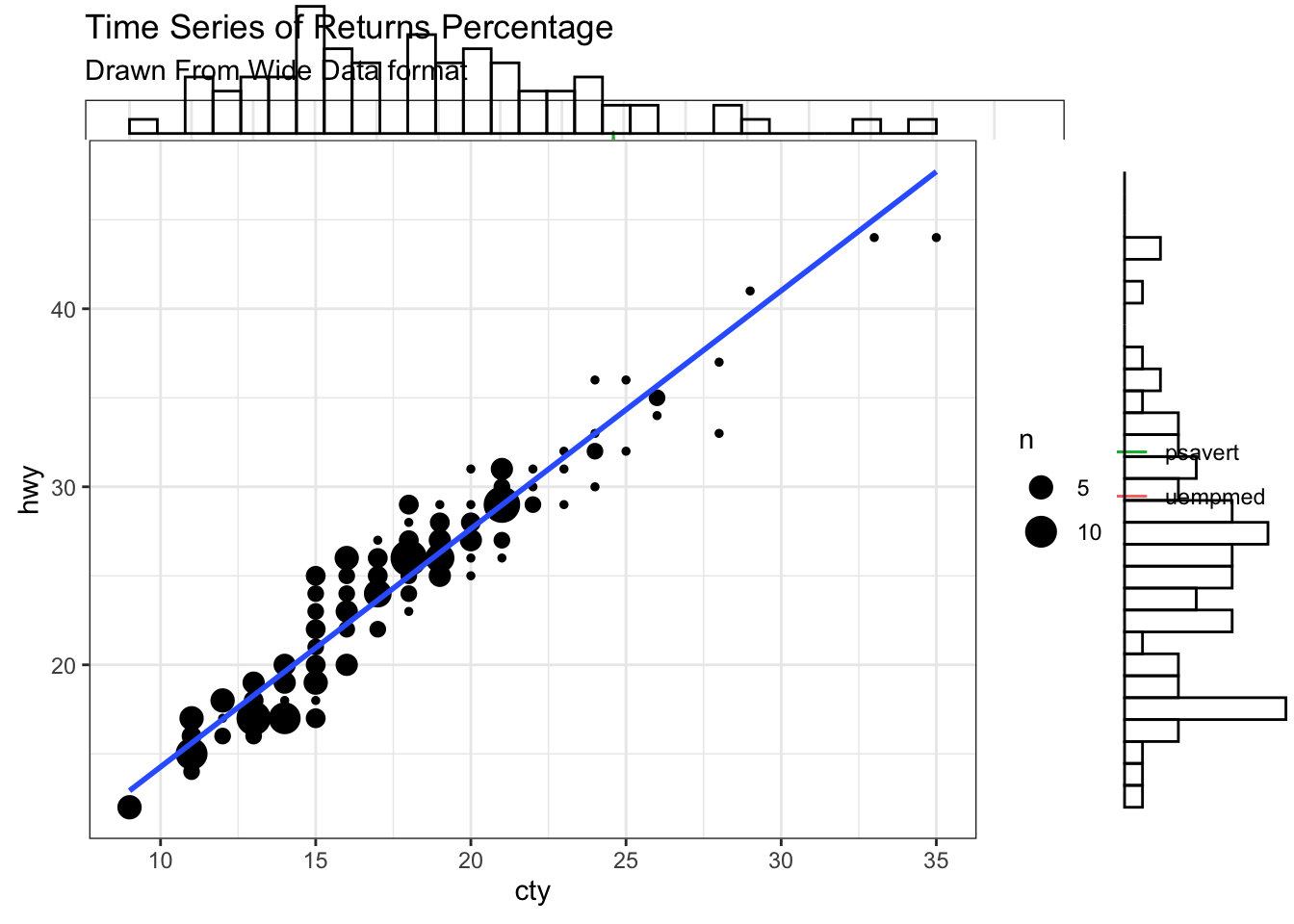# How to generate a table

``library(kableExtra)``
``## Warning: package 'kableExtra' was built under R version 3.6.2``
``````example <- read.csv("ozoneNA.csv")
example[1:4, c("WindDirection", "maxO3", "T9", "Ne9", "Vx15")] %>%
kbl() %>%
kable_styling()``````
WindDirection maxO3 T9 Ne9 Vx15
North 87 15.6 4 -0.6946
North 82 NA 5 -3.0000
East 92 15.3 2 0.5209
North 114 16.2 1 -0.1736

# Fancy plots seen in class

``library(ggplot2)``
``## Warning: package 'ggplot2' was built under R version 3.6.2``
``library(lubridate)``
``## Warning: package 'lubridate' was built under R version 3.6.2``
``````##
## Attaching package: 'lubridate'``````
``````## The following objects are masked from 'package:base':
##
##     date, intersect, setdiff, union``````
``````theme_set(theme_bw())

df <- economics[, c("date", "psavert", "uempmed")]
df <- df[lubridate::year(df\$date) %in% c(1967:1981), ]

# labels and breaks for X axis text
brks <- df\$date[seq(1, length(df\$date), 12)]
lbls <- lubridate::year(brks)

# plot
ggplot(df, aes(x=date)) +
geom_line(aes(y=psavert, col="psavert")) +
geom_line(aes(y=uempmed, col="uempmed")) +
labs(title="Time Series of Returns Percentage",
subtitle="Drawn From Wide Data format",
caption="Code's source: http://r-statistics.co/", y="Returns %") +  # title and caption
scale_x_date(labels = lbls, breaks = brks) +  # change to monthly ticks and labels
scale_color_manual(name="",
values = c("psavert"="#00ba38", "uempmed"="#f8766d")) +  # line color
theme(panel.grid.minor = element_blank())  # turn off minor grid

library(ggExtra)
data(mpg, package="ggplot2")

# Scatterplot
theme_set(theme_bw())  # pre-set the bw theme.
mpg_select <- mpg[mpg\$hwy >= 35 & mpg\$cty > 27, ]
g <- ggplot(mpg, aes(cty, hwy)) +
geom_count() +
geom_smooth(method="lm", se=F)

ggMarginal(g, type = "histogram", fill="transparent")``````
``## `geom_smooth()` using formula 'y ~ x'``
``## `geom_smooth()` using formula 'y ~ x'````````# ggMarginal(g, type = "density", fill="transparent")

library(ggthemes)
options(scipen = 999)  # turns of scientific notations like 1e+40

# X Axis Breaks and Labels
brks <- seq(-15000000, 15000000, 5000000)
lbls = paste0(as.character(c(seq(15, 0, -5), seq(5, 15, 5))), "m")

# Plot
ggplot(email_campaign_funnel, aes(x = Stage, y = Users, fill = Gender)) +   # Fill column
geom_bar(stat = "identity", width = .6) +   # draw the bars
scale_y_continuous(breaks = brks,   # Breaks
labels = lbls) + # Labels
coord_flip() +  # Flip axes
labs(title="Email Campaign Funnel") +
theme_tufte() +  # Tufte theme from ggfortify
theme(plot.title = element_text(hjust = .5),
axis.ticks = element_blank()) +   # Centre plot title
scale_fill_brewer(palette = "Dark2")  # Color palette``````